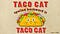# Palindrome Algorithm Explained using Random Stringfrom people.howstuffworks.com

A palindrome is a sequence (such as a word or combination of letters/numbers) that can read the same backwards and forwards. Writing a function which determines whether an input is a palindrome is one of the most common white-boarding question that an interviewer may ask.

There are many ways to write this algorithm and I will be showing you a way to check for palindromes in Javascript by using objects and a random string as our input.

Before we write our function, let’s think about problem step by step. We want to function to return true or false depending on the input to the function. To help visualize the function, I like to use examples. If we were to use “aabb” as an input, the function should return true. If the input is “aaab”, the function should return false. Next, pseudo-coding or writing comments can help break down how to write the function step by step.

To keep things simple, the input can only be lower case, no spaces, no numbers, and no empty strings.

It is not ideal to use in-built methods such as .reverse since our function will receive a random assortment of letters as our input. For example, the function will determine that “bob”, “obb” and “bbo” are all palindromes. To check if a random string is a palindrome, we need to keep track of how many occurrences each letter has. One way to do this is to create objects, storing the letter as the key and the number of occurrences as the value.

The first loop creates the key/value pairs for each letter. The variable obj creates an empty Object in which we will store the letter as the key and the number of occurrences as the value. The conditional inside the loop will either create a new key/value pair with the value set to 1 or increment the value if the key already exists.

The variable countValue is an array of the values from the variable obj. countValue is then iterated over to collect only the odd number of occurrences which is stored in the variable oddCount. Since a palindrome can only contain one or less than one number of odd occurrences, we can use a simple conditional to determine whether the input is a palindrome or not.

If we wanted to determine if a word is a palindrome, the function can be written much more concise using in-built functions.

This function will create an array of the string, reverse it, and join the elements in the array into a string. If the string is equal to the manipulated string, the function will return true. It is important to note that this does not work for strings with random letters.

## More from Jennifer Yoo

Software Engineer